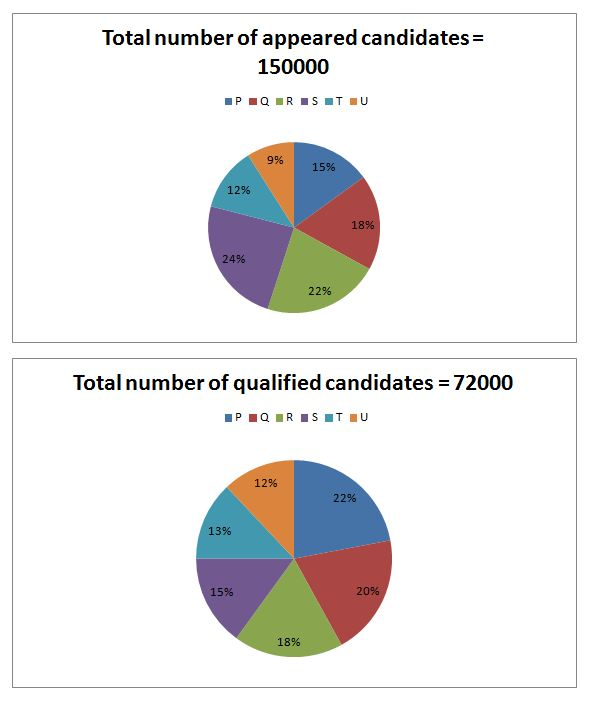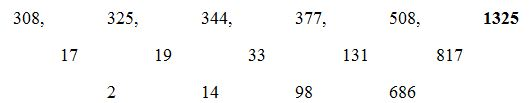# “20-20” Quantitative Aptitude | Crack IBPS RRB Assistant Prelims 2018 Day-174

Dear Readers, Find the aptitude test questions to crack latest bank exams. We regularly provide 20 aptitude test questions daily for students. Aspirants practice these questions on a regular basis to improve your score in aptitude section. Aspirants preparing for the exams can make use of this “20-20” Quantitative Aptitude Questions. Here we have started New Series of Practice Materials specially for IBPS RRB Assistant Prelims 2018. Aspirants those who are preparing for the exams can use this “20-20” Quantitative Aptitude Questions.

“20-20” Aptitude Questions | Crack IBPS RRB Assistant Prelims 2018 Day-174

Click “Start Quiz” to attend these Questions and view Solutions

Directions (Q. 1 – 5): What value should come in the place of question mark (?) in the following questions?

1) 34485 ÷ 11 + (5/9) of 12150 + 56 % of 9000 = ?2 – 451

a) 178

b) 216

c) 290

d) 124

e) None of these

2) (4858 + 6316 + 27578) ÷ 8 + 197 = (?)2

a) 52

b) 71

c) 63

d) 75

e) None of these

3) (75 % of 21200) ÷ ? = (210 % of 1200) × 5

a) 2 13/19

b) 3 17/23

c) 1 11/42

d) 3 3/4

e) None of these

4) (8/15) of 11130 + (4/9) of 2565 = ? × 4

a) 1769

b) 1552

c) 1478

d) 1957

e) None of these

5) 56 % of 3150 + (5/8) of 1960 – 54 % of 2800 = ?

a) 1423

b) 1576

c) 1288

d) 1354

e) None of these

Directions (Q. 6 – 10): What value should come in the place of question mark (?) in the following number series?

6) 72, 74, 151, 457, ?

a) 1527

b) 1245

c) 1833

d) 924

e) 1036

7) 308, 325, 344, 377, 508, ?

a) 1247

b) 1325

c) 1578

d) 1693

e) 2156

8) 27, 52, 231, 1280, ? , 82440

a) 8567

b) 8324

c) 8156

d) 9135

e) 9233

9) 729, 2071, 3081, 3819, ? , 4689

a) 4339

b) 4012

c) 4125

d) 4278

e) 4456

10) 256, 128, 192, 480, 1680, ?

a) 8120

b) 7560

c) 6650

d) 5680

e) 4340

11) The length and breadth of a rectangular piece of land are in the ratio of 7 : 3. The owner spent Rs. 3600 for surrounding it from all the sides at the rate of Rs. 6 per meter. The difference between length and breadth is?

a) 50 m

b) 100 m

c) 200 m

d) 120 m

e) None of these

12) A box contains 6 pink, 4 red and 5 yellow marbles. If 2 marbles are picked at random, then find the probability that the marbles are either yellow or pink?

a) 9/31

b) 11/34

c) 15/29

d) 5/21

e) None of these

13) Ravi has 35 % savings with him from his monthly salary. If the expenditure of clothing is 20 % of overall expenditure and his expenditure except clothing is Rs. 10400. Find his savings?

a) Rs. 6500

b) Rs. 5850

c) Rs. 7000

d) Rs. 7150

e) None of these

14) The difference between the two numbers is 3240. 52 % of one number is equal to 25 % of another number. Find the two numbers?

a) 3000, 6240

b) 4500, 5680

c) 3660, 4840

d) 5250, 6430

e) None of these

15) Raju is as much older than Moni as he is younger than Anu. If the sum of the ages of Anu and Moni is 56 years, then how old is Raju?

a) 28 years

b) 25 years

c) 22 years

d) 18 years

e) None of these

Directions (Q. 16 – 20) Study the following graph carefully and answer the given questions:

Following pie chart 1 shows the total number of candidates appeared for NEET examination from different states and pie chart 2 shows the total number of candidates qualified for NEET examination.16) Find the ratio between the total number of qualified candidates from State P and R together to that of total number of appeared candidates from State Q?

a) 56 : 51

b) 45 : 42

c) 16 : 15

d) 66 : 61

e) None of these

17) Find the average number of appeared candidates from State P, R and T together?

a) 24500

b) 26800

c) 21500

d) 22700

e) None of these

18) Find the difference between the total number of qualified candidates from State Q and S together to that of total number of qualified candidates from State T and U together?

a) 6500

b) 7200

c) 5600

d) 5000

e) 6800

19) Total number of appeared candidates from state S is approximately what percentage of total number of qualified candidates from State P?

a) 180 %

b) 230 %

c) 200 %

d) 150 %

e) 120 %

20) Total number of qualified candidates from State R is what percentage more/less than the total number of qualified candidates from State T?

a) 25 % less

b) 50 % more

c) 40 % more

d) 15 % less

e) 35 % less

Direction (1-5):

(34485/11) + (5/9)*12150 + (56/100)*9000 = x2 – 451

3135 + 6750 + 5040 + 451 = x2

15376 = x2

X = 124

(4858 + 6316 + 27578) ÷ 8 + 197 = (?)2

38752/8 + 197 = x2

4844 + 197 = x2

5041= x2

X = 71

[(75/100)*21200]/x = [(210/100)*1200]*5

15900/x = 12600

X = 15900/12600

X = 53/42 = 1 11/42

(8/15)* 11130 + (4/9)* 2565 = 4x

4x = 5936 + 1140

4x = 7076

X = 7076/4 = 1769

(56/100)*3150 + (5/8)*1960 – (54/100)*2800 = x

X = 1764 + 1225 – 1512

X = 1477

Direction (6-10):

The pattern is, *1 + 2, *2 + 3, *3 + 4, *4 + 5, *5 + 6,..The difference of difference is, *7

The pattern is, *1 + 25, *3 + 75, *5 + 125, *7 + 175, * 9 + 225,..

The pattern is, + (113 + 11), + (103+ 10), + (93 + 9), + (83 + 8), + (73 + 7),..

The pattern is, *1/2, *3/2, *5/2, *7/2, *9/2,..

Perimeter of the field = 3600/6 = 600 m

2*(7x + 3x) = 600 => x = 30

So, length = 210 m  and breadth = 90 m

= > (210 – 90) m = 120 m

Total probability = 15C2

Required probability = 5C2 or 6C2

The probability that the marbles are either red or pink,

= > 5C2 or 6C2 / 11C2

= > 5/21

Savings = 35 %

Expenditure = 65 %

According to the question,

= > (80/100)*expenditure = 10400

Expenditure = 13000

(65/100)*monthly salary = 13000

Monthly salary = 13000*(100/65) = Rs. 20000

Savings = 35 % of monthly salary = (35/100)*20000

= > Rs. 7000

Let the two numbers be x and y,

X – Y = 1180

(52/100)*x = (25/100)*y

(X/Y) = 25/52

27’s = 3240

1’s = 120

The two numbers be,

= > 25’s = (120*25) = 3000

= > 52’s = (120*52) = 6240

Raju – Moni = Anu – Raju

2* Raju = Anu + Moni

Sum of the ages of Moni and Anu = 56 years

2*Raju = 56

Raju = 56/2 = 28 years

Direction (16-20):

The total number of qualified candidates from State P and R together

= > 72000*(40/100) = 28800

The total number of appeared candidates from State Q

= > 150000*(18/100) = 27000

Required ratio = 28800 : 27000 = 16: 15

The average number of appeared candidates from State P, R and T together

= > 150000*[(15 + 22 + 12)/100]*(1/3) = 24500

The total number of qualified candidates from State Q and S together

= > 72000*(35/100) = 25200

The total number of qualified candidates from State T and U together

= > 72000*(25/100) = 18000

Required difference = 25200 – 18000 = 7200

Total number of appeared candidates from state S

= > 150000*(24/100) = 36000

Total number of qualified candidates from State P

= > 72000*(22/100) = 15840

Required % = (36000/15840)*100 = 227 % = 230 %